# lab3 .pdf

### File information

This PDF 1.5 document has been generated by TeX / MiKTeX pdfTeX-1.40.15, and has been sent on pdf-archive.com on 02/03/2016 at 22:53, from IP address 128.62.x.x. The current document download page has been viewed 966 times.
File size: 158.48 KB (10 pages).
Privacy: public file

### Document preview

Lab 3: Generating Assembly Code
CS 429: Spring 2016
Assigned: March 2, 2016
Due: March 23, 2016
Last updated: March 1, 2016 at 16:01

1

Introduction

Note: Mike Feilbach (mfeilbach@utexas.edu) is the lead TA for this lab. Please see Mike for
detailed or specific questions about Lab 3.
This lab is designed to give you experience in C programming and a bit of experience with
x86-64 assembly. You will be writing a very basic compiler—in other words, a program that
produces x86-64 assembly code.
Your program will take in a reverse Polish notation (RPN) arithmetic expression and produce
assembly code for a function that computes the value of that expression. This will be explained
in detail later in this document.

2

Logistics

This is an individual project. You may not share your work on lab assignments with other
students, but feel free to ask instructors for help if you are stuck (e.g., during office hours or
discussion section).
You will turn in a tarball (a .tar.gz file) containing the source code for your program, written in
C. You must include a Makefile, such that we can build your code using the make command.
(If you did Lab 0, you are already familiar with writing makefiles.) Ensure that your code
compiles and runs correctly on skipper.cs.utexas.edu (I’m just choosing a fixed machine for
consistency).
Any clarifications or corrections for this lab will be posted on Canvas or Piazza, and if necessary,
fixes will be committed to this file.

3

Background

Reverse Polish notation (RPN) is an alternative way to represent arithmetic expressions like
those you might type into a calculator. At one point in time, it was popular to use RPN in
physical calculators because it was easier to implement a calculator that read RPN as input.
Similarly, it will be easier for you to do this assignment with RPN than with the normal style
of writing arithmetic expressions.
In RPN, arithmetic operators such as +, *, and - come after their two operands, rather than
in between them. Here are some examples of normal notation vs. RPN.

CS429: Programming Assignment 3

2

“Normal” Notation
(1 + 1)
(3 * (4 + 7))
(((8 + 9) * (4 + (3 * 10))) - 5)

RPN
1 1 +
3 4 7 + *
8 9 + 4 3 10 * + * 5 -

Notice that parentheses are not required to write expressions in RPN. Since each operator
has a fixed number of arguments (arity) of two, the design of a calculator with RPN input is
simplified. For example, nested parentheses don’t need to be kept track of. In fact, a RPN
calculator can be implemented with a stack data structure using two very simple rules.
A RPN calculator operates on a token-by-token basis, where each token is either a number or
an operator. The input is a sequence of tokens, and one token is parsed at a time. The following
two rules govern how a sequence of tokens describing an expression is evaluated.
1. When a number n is parsed, the calculator pushes n on its stack.
2. When an operator is parsed, the calculator pops two numbers off the stack (y is from
the first pop, x from the second pop), performs the operation z = (x y), then pushes z
on its stack.
Here is an example of how a RPN calculator processes the last example from above. The left
column is what the stack looks like after parsing each token listed in the right column. In this
diagram, the bottom of the stack is on the left hand side.
8
8 9
17
17 4
17 4 3
17 4 3 10
17 4 30
17 34
578
578 5
573

Token
Token
Token
Token
Token
Token
Token
Token
Token
Token
Token

parsed:
parsed:
parsed:
parsed:
parsed:
parsed:
parsed:
parsed:
parsed:
parsed:
parsed:

8
9
+
4
3
10
*
+
*
5
-

=&gt;
=&gt;
=&gt;
=&gt;
=&gt;
=&gt;
=&gt;
=&gt;
=&gt;
=&gt;
=&gt;

Push 8.
Push 9.
Perform (8 + 9), push the result.
Push 4.
Push 3.
Push 10.
Perform (3 * 10), push the result.
Perform (4 + 30), push the result.
Perform (17 * 24), push the result.
Push 5.
Perform (578 - 5), push the result.

Explanation: The first token parsed is 8. This token is a number, therefore it is pushed on
the stack. The second token is 9. This token is a number, therefore it is pushed on the stack.
The third token is +. Therefore, two numbers are popped from the stack: 9 from the first pop
and 8 from the second pop. 8 + 9 is computed, and the result (17) is pushed on the stack.
This pattern continues until the expression calculation is completed or an error occurs. Errors
and other conditions are described later on.

CS429: Programming Assignment 3

4

3

The RPN compiler

We define in this section a simple compiler that takes an expression via the command line and
dumps assembly code to stdout (standard output). The assembly code will entail a function
called compute that accepts as arguments three values for the variables x, y, and z respectively,
and returns the result of the expression.
The input to your program is a RPN expression (sequence of tokens) given on the command
line. This expression may contain any number of integer constants, the variables x, y, and z,
and any number of operators. Integer constants may be positive, zero, or negative, meaning
that they will consist of numeric digits, possibly with a minus sign at the beginning. The
following operators are allowed: +, *, - (plus, times, minus). Expressions are written in RPN,
as described earlier.
A few sample invocations of your program might be:
\$ .\compiler x
[some assembly
\$ .\compiler x
[some assembly

4 + 2 "*" 4 code is printed here]
4 + 2 4 "*" code is printed here]

Note: In most shells (including bash, the one you are probably using), the * character is
used to mean “all the files in the current directory,” so unfortunately, the * needs to be put in
quotation marks for our purposes, otherwise the shell will replace it with a list of all the files
in the current directory.
As an example, if variable x has value 3, these expressions should equal 10 and -1, respectively.
If variable x has value 4, the expressions should equal 12 and 0, respectively.
Therefore, the assembly code produced by the first run of your compiler should be a function
that returns the number 10 when called with argument x set to 3, and the number 12 when
called with argument x set to 4. The assembly code produced by the second run should be a
function that returns the number -1 with argument x set to 3, and the number 0 with argument
x set to 4.
The output of your program will be x86-64 assembly code, printed to stdout (standard output)
as shown above. This code should contain a function named compute which accepts three
arguments (x, y, and z) according to the standard x86-64 calling convention. compute should
be designed to return the value of the input RPN expression where the values of x, y, and z
have been substituted in. More on that below.
First, think about how RPN is evaluated in a bit more detail. Given an RPN expression, do
the following, parsing one token (command line argument) at a time:
1. If you encounter a variable, push its value on the runtime stack.
2. If you encounter a constant, push it on the runtime stack.

CS429: Programming Assignment 3

4

3. If you encounter an operator, pop the right number of arguments (i.e., two), apply the
operator, and push the result on the runtime stack.
4. If there is a single numeric value on the runtime stack and the input is exhausted, then
this is the answer to the expression, so simply pop and return it.
Note that we are directly using “the stack” as our RPN calculation stack, rather than making
a separate stack data structure.
Various problems might occur while evaluating an expression. For example,
1. An unrecognizable symbol is encountered (i.e., something other than +, -, or *).
2. There may not be enough arguments on the stack for the operator you are attempting to
apply.
3. There may be more than one value left on the stack when you are finished (i.e., when the
input is exhausted).
To help identify some of these situations, maintain an integer variable to keep track of how
many values are on currently on the stack when seeing if the expression is valid in your C code.
If you encounter any of these errors, print a useful error message to stderr, not stdout, and
terminate with a nonzero exit code. Examples of illegal inputs are:
• a (because the variable a is not allowed—only x, y, and z are allowed)
• 4 + (because + needs two arguments)
• 3 4 (because it will leave two values on the stack)
• 3 4 4 2 + (because it will leave three values on the stack)
• + (because + needs two arguments)
Aside: It’s important to print to stderr rather than stdout so the user can separate the
assembly code output from any error messages produced. For example, when redirecting stdout
to a file using the special shell directive “&gt;”, stderr messages are still printed to the screen,
while the assembly code output goes to the file specified. Sample invocations are listed below.
\$ ./compiler
\$ ./compiler
\$ ./compiler
Error: The *
\$ ./compiler

1 2 3 4 + + + &gt; output1.s
1 2 3 4 "*" - 5 6 7 + + - "*" 8 9 "*" + &gt; output2.s
2 "*" 2 &gt; output3.s
operator requires two arguments
2 2 "*" &gt; output4.s

CS429: Programming Assignment 3

5

In all four invocations, any assembly instructions printed to stdout will be silently put in the
specified files (i.e., output1.s, output2.s, output3.s, and output4.s), which we are naming
with the .s extension because .s is used as the extension for assembly code files. Notice in the
third invocation there is an error message. The error message didn’t get sent to the output3.s
file, but rather to the screen, because “&gt;” only redirects stdout, not stderr.
Note that if an expression is not legal, assembly output should not be given.
Now, imagine parsing a legal RPN expression and generating an x86-64 subroutine that can
evaluate it. Our expressions may contain only variables x, y, and z. We assume that these are
passed (in order) to your program via the usual calling mechanism. That is, x, y, and z are
passed in the registers %rdi, %rsi, and %rdx, respectively. You should return your answer in
register %rax, which again is specified by the standard calling conventions.
Consider the following RPN expression:
x 4 + 2 * 4 A first attempt at compiling this might be to generate the following sequence of x86-64 instructions (probably without the comments):
.globl compute

compute:
pushq %rdi
pushq \$4
popq %r10
popq %r11
pushq %r11
pushq \$2
popq %r10
popq %r11
imulq %r10, %r11
pushq %r11
pushq \$4
popq %r10
popq %r11
subq %r10, %r11
pushq %r11
popq %rax
retq

# Note: this marks that the label
# "compute:" is a function name
# that can be used from other code.
#
#
#
#
#
#
#
#
#
#
#
#
#
#
#
#
#
#

push x on the stack
push 4 on the stack
. add the top two numbers
|
|
push the result
push 2 on the stack
. multiply the top two numbers
|
|
push the result
put 4 on the stack
. subtract the top two numbers
|
|
push the result
pop final answer into the return register
return from the function

However, this is inefficient for a few reasons. First, numbers are pushed on the stack and
immediately popped off into registers. Second, numbers are being popped off the stack into

CS429: Programming Assignment 3

6

registers before performing arithmetic operations on them. It’s true that in x86-64, we can’t
write arithmetic instructions where both the source and destination are in memory, however
sometimes it is possible to operate on a value that is on the stack when using an integer constant.
This can be done with some instructions (like add), but not others (like imul).
Here is a second attempt, with some optimizations added:
.globl compute
compute:
pushq %rdi
popq %r8
imulq \$2, %r8
pushq %r8
subq \$4, (%rsp)
popq %rax
retq

#
#
#
#
#
#
#
#

push x on the stack
. multiply by 2 (using a temporary
| register)
push the result
subtract 4 (directly in memory)
pop final answer into the return register
return from the function

Notice that add and sub can target a memory location, but imul must target a register. Experiment with (or search the web for information about) different instructions to see which ones
can target a memory location and which ones have to use registers.
You can use the as command to call the GNU assembler on your assembly code to check if it
is valid x86-64 assembly. Use the --64 flag to ensure you’re assembling as x86-64, as opposed
to 32-bit x86 or any other kind of assembly code.
Now, there are even more optimizations we might be able to do. Notice how in the previous
assembly code, we never put more than one thing on the stack at a time. So why don’t we just
use a register for that one location on the stack we keep using? Then we don’t need to access
memory at all. In fact, we can just use the %rax register, which we eventually return.
Here’s a third attempt:
.globl compute
compute:
movq %rdi, %rax
imulq \$2, %rax
subq \$4, %rax
retq

#
#
#
#
#

put x in %rax
multiply %rax by 2
subtract 4 from %rax
return from the function

Note that we’ve started doing optimizations that are specific to the exact expression we started
out with, x 4 + 2 * 4 -. These particular optimizations will not work for all expressions.
Therefore, if you want to implement these optimizations, the C code which generates this
assembly will have to be smart and know when it can and can’t do certain optimizations.
For your compilation, do not use callee saved registers. In other words, only use the following
registers:

CS429: Programming Assignment 3

7

• %rax: for the return value and possibly for intermediate storage
• %rsp: the stack pointer
• %rdi, %rsi, and %rdx: the registers used for passing in the three arguments
• %rcx, %r8, and %r9: the three unused argument-passing registers
• %r10, and %r11: the other two caller-saved registers
You can reuse registers if it’s safe to do so, and you can push things onto the stack if you run
out of registers at any point.
Your code should be able to handle arbitrary RPN expressions that follow our constraints on
variables.

5

You don’t want to submit your code without testing it first. In this section various ways in
which you can make sure your code is working correctly are covered.

5.1

Make sure your C code doesn’t have any syntax errors, etc., and that your Makefile is in order.
You should be able to go to the directory where your code is, type make, and have an executable
file called compiler be created in the directory. Test this on skipper.cs.utexas.edu—this
machine has an environment similar to what we’ll use for grading.

5.2

Your C program should produce valid x86-64 assembly code

Make sure that when you run the compiler executable which you created with a valid RPN
expression (passed in as a series of command line arguments), valid assembly code is printed to
the screen.
Try some of the example invocations mentioned earlier in this document, and make up some
Beyond just eyeballing it, make sure that the output is valid assembly code by using the special
shell directive “&gt;” (as mentioned earlier) to make your program direct its output into a .s file
such as test.s, and then run the assembler on it to see if there are any syntax errors or other
problems:
\$ as --64 test.s
(As mentioned before, the --64 is to ensure that the assembly code is treated as x86-64 assembly
code.)

CS429: Programming Assignment 3

5.3

8

The assembly code should provide the right function

Your program is required to produce assembly code for a function called compute which takes
three 64-bit signed integer arguments and returns a 64-bit signed integer argument. In C, that
means the function would have looked something like this:
#include &lt;stdint.h&gt; // this needs to be at the top of the file
int64_t compute (int64_t x, int64_t y, int64_t z) {
// do stuff
return something;
}
(In practice you’d often write long long rather than int64_t, and then you wouldn’t need the
#include &lt;stdint.h&gt;, but unlike long long, the int64_t type is guaranteed to be exactly
64 bits long, no matter what compiler or architecture you use.)
Since you’re writing the function in assembly, if you wanted to use it in some C code, you
must “import” it. This is how to do it in C: first, somewhere in your C code, make an extern
declaration of the function compute, like so:
#include &lt;stdint.h&gt; // this needs to be at the top of the file
extern int64_t compute (int64_t x, int64_t y, int64_t z);
Notice that no function body is provided, only the argument types, return type, and name of the
function, with the word extern (for “externally defined”). Then, when you compile your C code,
specify the name of the assembly file which contains the function compute, and gcc will hook it
up for you. For example, assuming the assembly code for compute is in compute function.s,
\$ gcc -o full_program some_program.c compute_function.s
So, to test that your assembly code provides the correct function, write a C program which
uses the compute function you defined (i.e., it calls compute on some arguments and prints the
result). Make sure to put in the extern definition shown above.
Then, compile the program, telling gcc the name of the file where you saved the assembly code
output from your compiler. If there are no errors, then gcc was able to read your assembly
code as a definition of a compute function, as desired. (If you follow the format shown in the
assembly code examples above, you shouldn’t have any problems.)
By the way, if you’re interested in how this linking process works, check out Chapter 7 in the
textbook, which we may get to later in the semester.

CS429: Programming Assignment 3

5.4

9

The generated functions should work correctly

Try compiling a few RPN expressions of your own choosing. For each one, your program will
produce some assembly code. Create a C program that will link with your assembly code (as
described in the previous section) and test it by calling it with some particular values of x, y,
and z and printing the result. Compile the test program together with the assembly code and
run the resulting executable to see if it prints the number you expected.
Try various different kinds of RPN expressions to make sure you did everything correctly. Try
some with many operators, some with many numbers in a row, some with negative numbers,
etc.
If you’re feeling really ambitious, you might try to write a randomizing test harness that automatically tests your compiler on many different randomly generated RPN expressions, and
substitutes in many different randomly generated values of x, y, and z for each RPN expression.

5.5

Your submission should be in the correct format

Once you’ve finished programming, it’s time to submit your work. Create a README file
explaining the optimization tricks you used to make your assembly code faster, if
any, and then use the tar utility to create an archive (“tarball”) of the directory in which you’ve
created your code. This tarball should be named: &lt;lastName&gt; &lt;firstName&gt; &lt;UT-eid&gt; lab3.tar.gz.
The tarball should only contain source code, so get rid of any compiled files, generated assembly
files, and any other files before making the tarball. We should be able to type make after
extracting your tarball and get a working compiled version of your code, which we
will test. Please make sure this is the case, because if there are errors using make
this works on skipper.cs.utexas.edu, as mentioned earlier.)

6

Your assignment is to write a compiler program as described above.
The basic grading policy for this lab is that you’ll get 100 points (full normal points) if
you create a program that correctly does what it is supposed to do—that is, accepts RPN
expressions on the command line and dumps assembly code to stdout which defines a function
called compute that takes arguments x, y, and z and returns the value of the RPN expression
when those values are substituted in for the variables x, y, and z in the expression.
If you produce straightforward (but inefficient) assembly code such as that shown in the first
example, you will still earn the 100 points (full points) if the code behaves correctly.
Up to 10 extra credit points are possible if you optimize the assembly code you generate in
some significant way. Two such ways are as follows.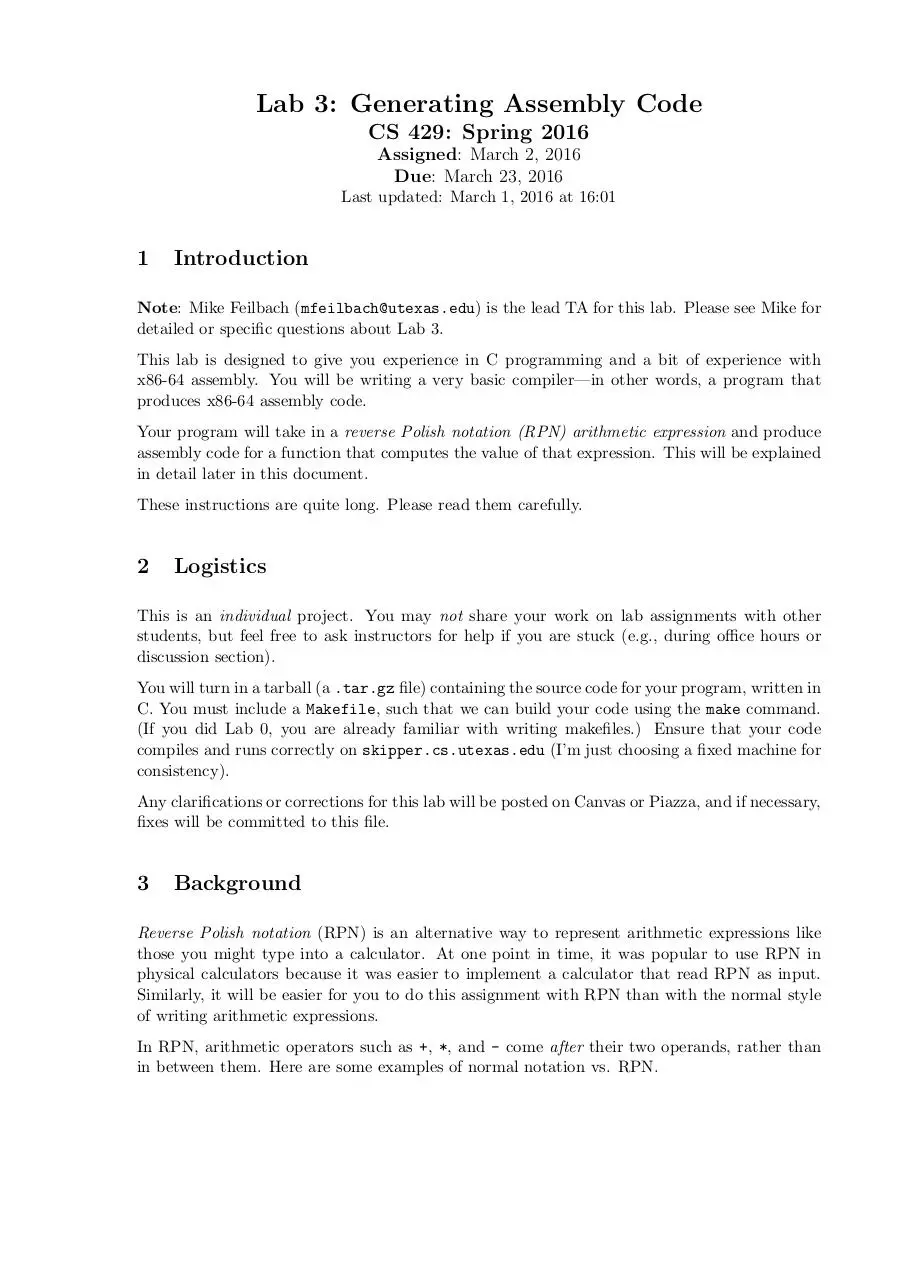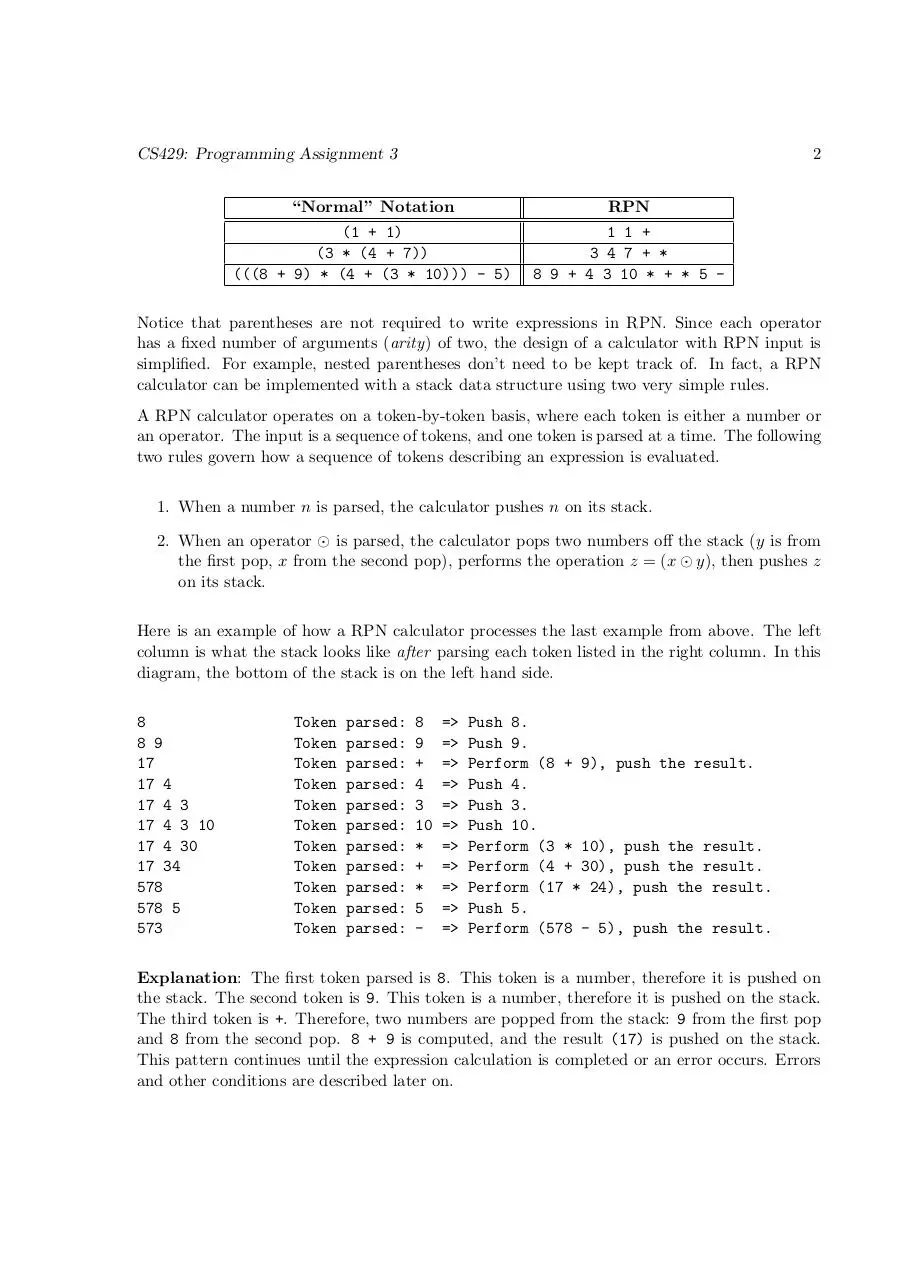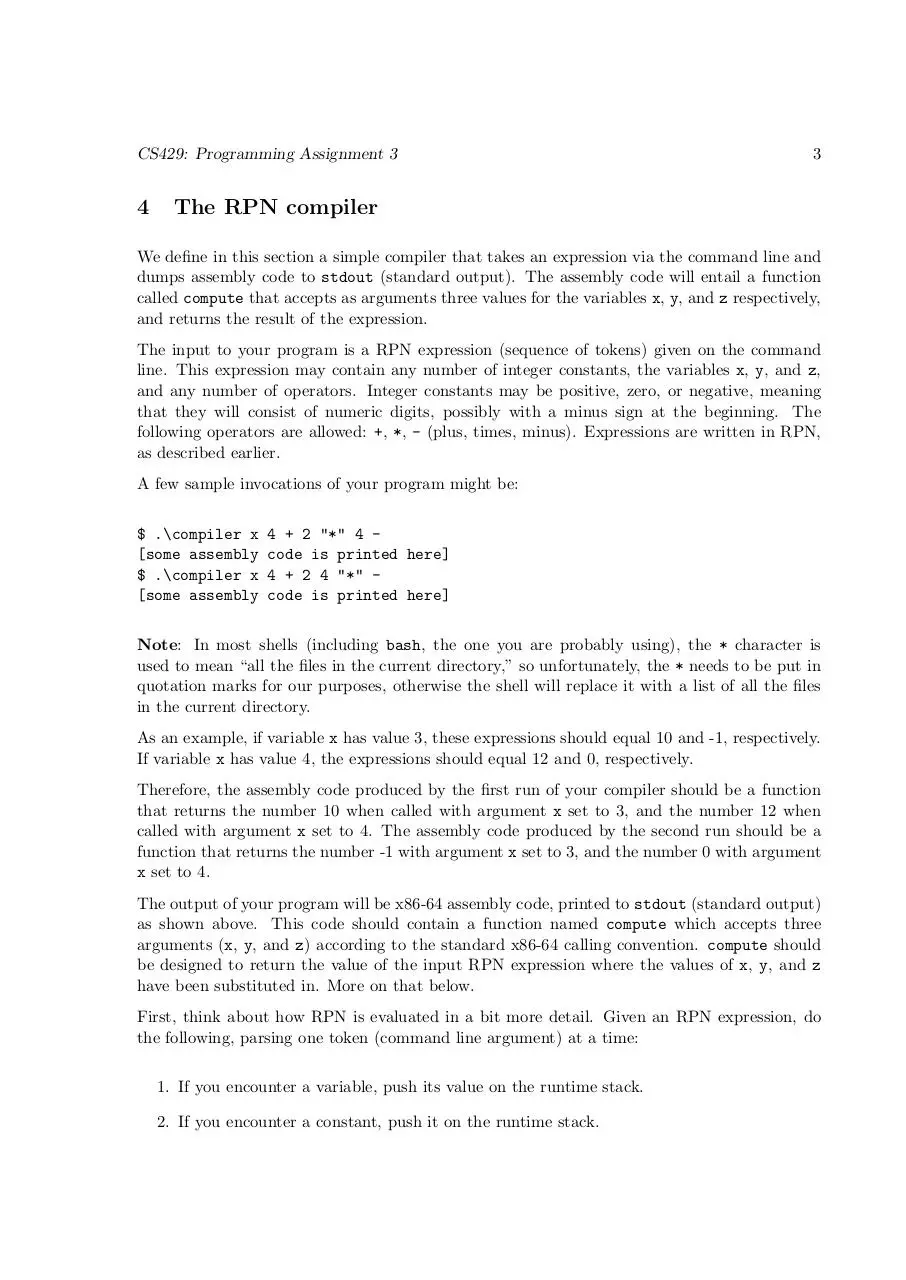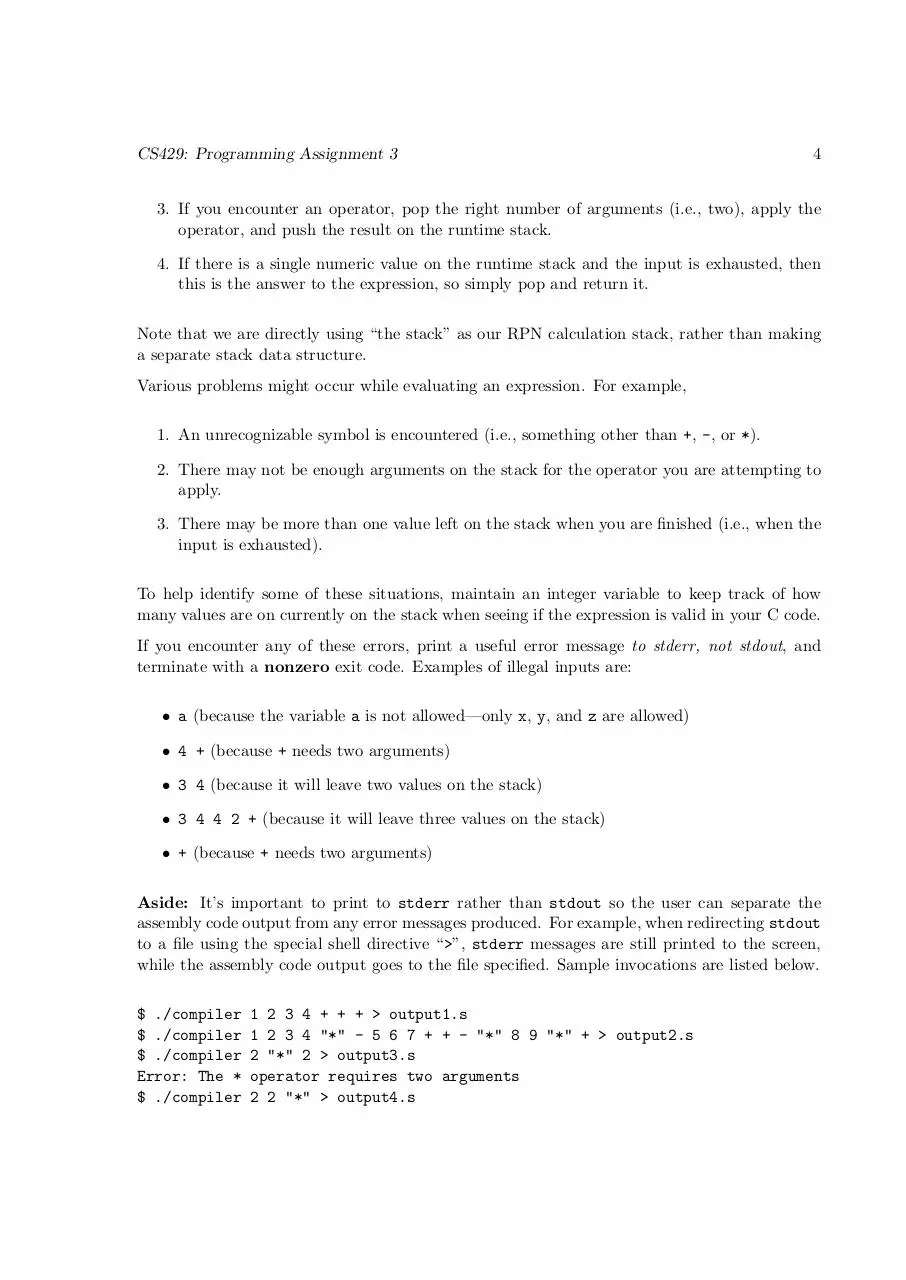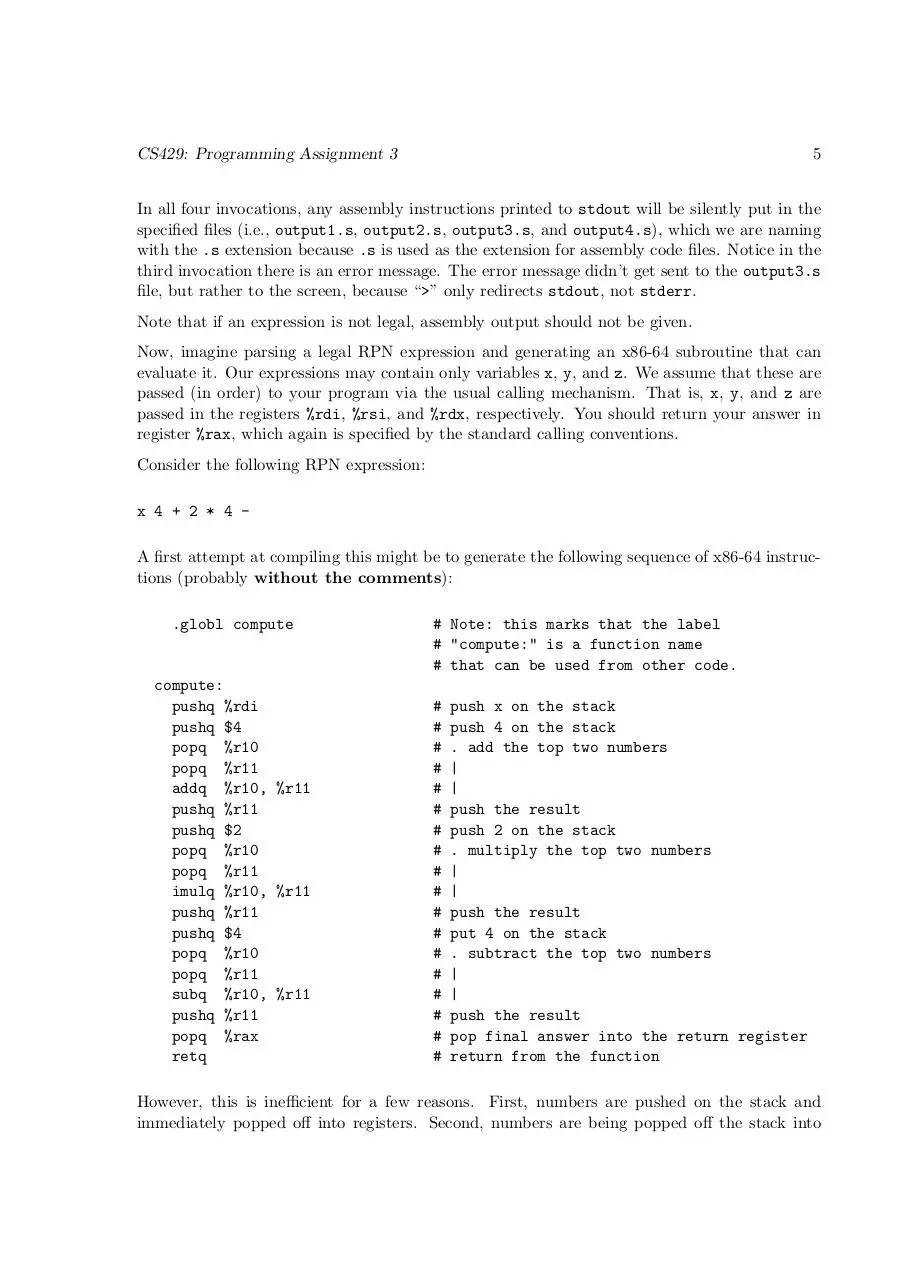lab3.pdf (PDF, 158.48 KB)

### Share on social networks

#### HTML Code

Copy the following HTML code to share your document on a Website or Blog